### 3. 实验任务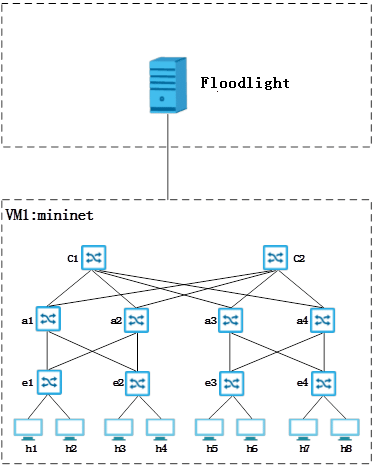### 4.实验步骤

#### 4.1启动Floodlight

java -jar target/floodlight.jar

#### 4.2创建数据中心

cd /home/mininet

touch fattree.py

vim fattree.py

```#!/usr/bin/python
#创建网络拓扑
"""Custom topology example
Adding the 'topos' dict with a key/value pair to generate our newly defined
topology enables one to pass in '--topo=mytopo' from the command line.
"""
from mininet.topo import Topo
from mininet.net import Mininet
from mininet.node import RemoteController,CPULimitedHost
from mininet.util import dumpNodeConnections

class MyTopo( Topo ):
"Simple topology example."

def __init__( self ):
"Create custom topo."

# Initialize topology
Topo.__init__( self )
L1 = 2
L2 = L1 * 2
L3 = L2
c = []
a = []
e = []

for i in range( L1 ):
sw = self.addSwitch( 'c{}'.format( i + 1 ) )
c.append( sw )

for i in range( L2 ):
sw = self.addSwitch( 'a{}'.format( L1 + i + 1 ) )
a.append( sw )

for i in range( L3 ):
sw = self.addSwitch( 'e{}'.format( L1 + L2 + i + 1 ) )
e.append( sw )

for i in range( L1 ):
sw1 = c[i]
for sw2 in a[i/2::L1/2]:

for i in range( 0, L2, 2 ):
for sw1 in a[i:i+2]:
for sw2 in e[i:i+2]:

count = 1
for sw1 in e:
for i in range(2):
host = self.addHost( 'h{}'.format( count ) )
count += 1
topos = { 'mytopo': ( lambda: MyTopo() ) }```

screen mn --custom /home/mininet/fattree.py --topo mytopo --controller=remote,ip=10.0.0.20,port=6633 --switch ovsk,protocols=OpenFlow10

mn为mininet启动命令。

--mac指定虚拟主机的mac地址顺序编号，若不带此参数则随机编号

--controller指定of交换机的控制器

--switch指定虚拟交换机的类型，ovsk表示虚拟交换机为ovs Kernel mode

--custom指定自定义拓扑文件

--topo指定加载拓扑的名字

#### 4.3多数据中心主机连通性及通信带宽测试

4.3.1同一交换机内部的主机间连通性及通信带宽测试

4.3.2相同汇聚交换机下不同机架的主机间测试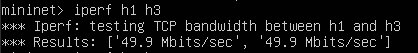4.3.3相同核心交换机不同汇聚交换机下的主机间测试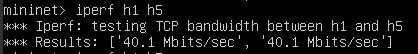4.3.4所有主机的连通性验证及带宽测试

```#在开头补充
from mininet.log import setLogLevel
#测试连通性代码部分
def simpleTest():
"Create and test a simple network"
topo = MyTopo()
net.addController( 'c0', controller=RemoteController, ip='10.0.0.20', port=6633 )
net.start()
print "Dumping host connections"
dumpNodeConnections(net.hosts )
print "Testing network connectivity"
net.pingAll()
print "Testing bandwidth between h1 with h2, h3, h5"
h1, h2 = net.get( 'h1', 'h2' )
net.iperf( ( h1, h2 ) )
h1, h3 = net.get( 'h1', 'h3' )
net.iperf( ( h1, h3 ) )
h1, h5 = net.get( 'h1', 'h5' )
net.iperf( ( h1, h5 ) )
net.stop()

if __name__ == '__main__':
# Tellmininet to print useful information
setLogLevel( 'info' )
simpleTest()```

net.addController( 'c0', controller=RemoteController, ip='10.0.0.20', port=6633 )

sudo ./fullfattree.py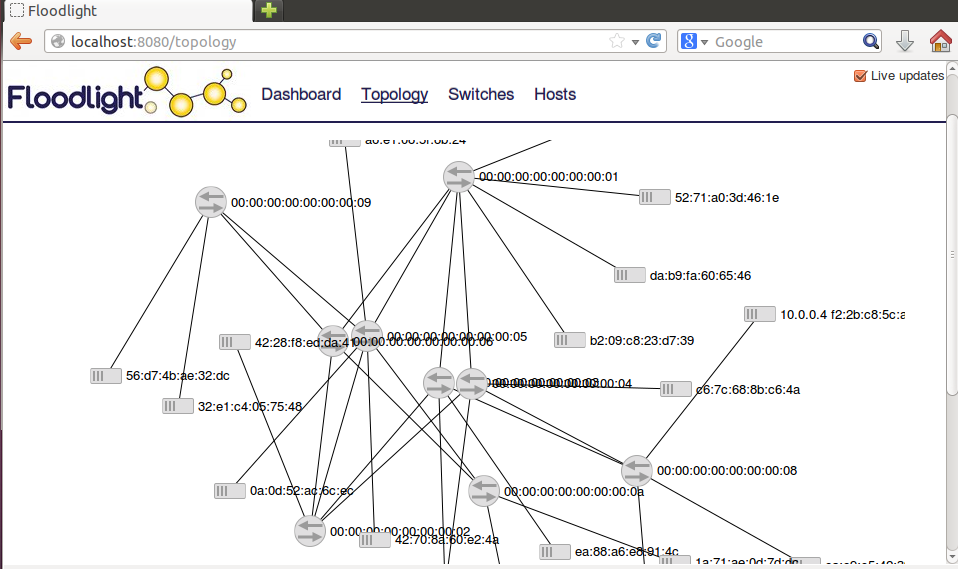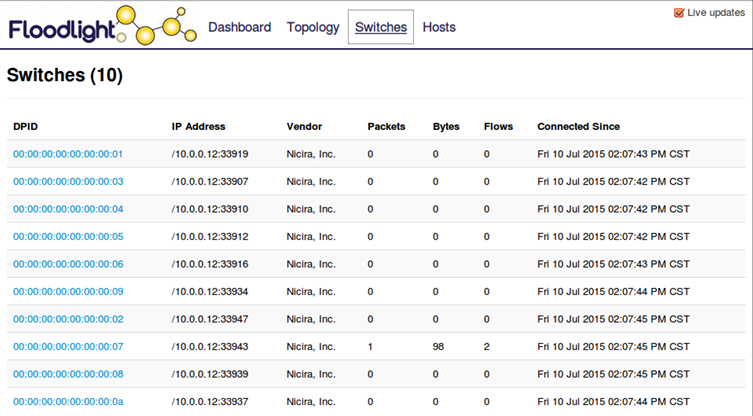### 5.实验结论

Mininet创建网络拓扑的代码中，可以通过改变代码中定义的L1变量来设置核心交换机的数量，并通过添加额外的交换机和链路来构成更复杂的数据中心网络拓扑。随着边缘交换机的增加，主机个数也随之增长，利用Mininet的易用性和扩展性，可以创建基于多种数据中心场景下的网络拓扑，达到更好更全面的实验效果。